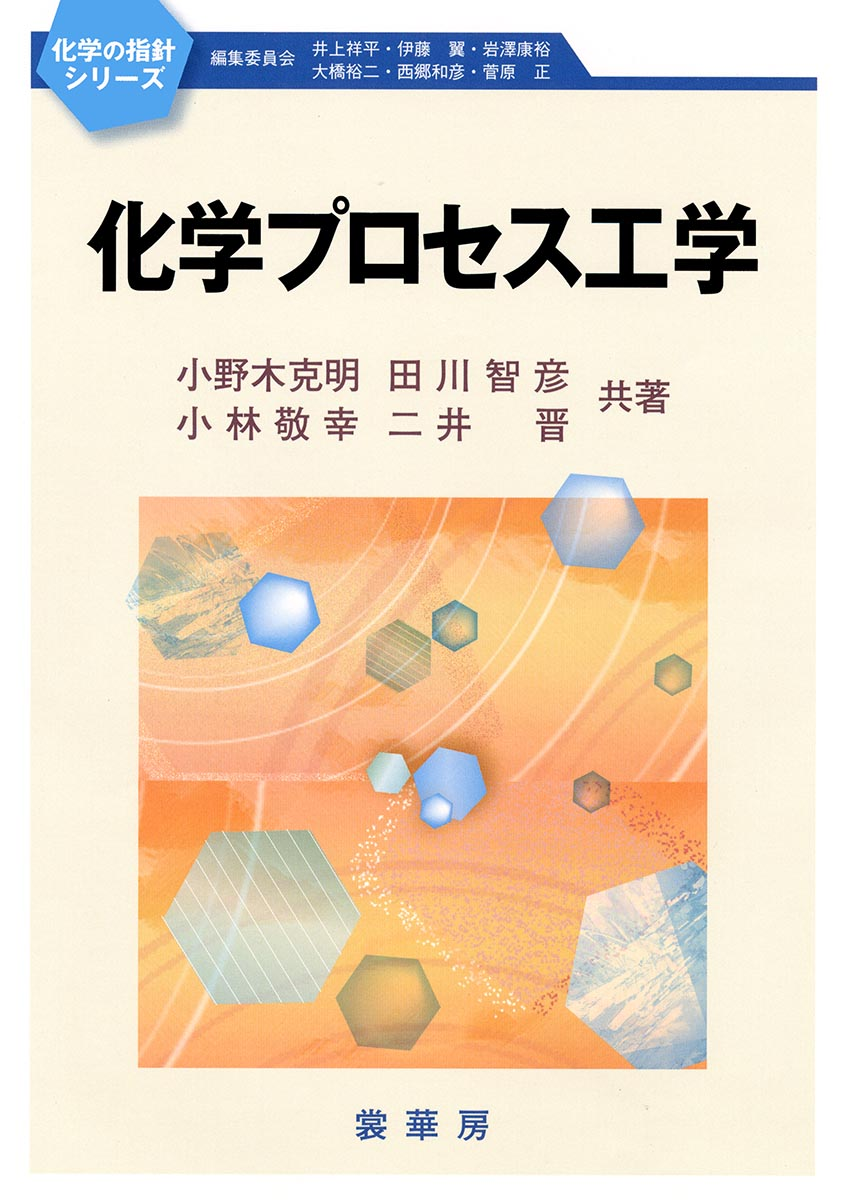PR

# Formula for calculating the required stirring power

This section explains the calculation formula for stirring power.

We’ll focus on the basics that users who use many stirrers should know.

There are formulas for the power required for stirring that are well-known in the industry, such as Nagata’s formula, but they are quite specialized.

If you are not used to working with expressions, this can be quite confusing.

I used this formula when I was a new employee, but I remember taking a detour because I couldn’t understand the essence of it.

I would like to explain the basics so that this does not happen.

## Stirring power system

We will explain the model necessary to consider the power required for stirring.

First of all, there were many explanations that jumped into detailed formulas without explaining the system, which I also had a hard time with.

When thinking about using electricity to operate a stirrer, we need two things: electricity and machinery.

It’s the same story with the pump electric motor.

For pumps, there are many mechanical engineers who focus on the mechanical part of calculating pressure loss and ignore the electrical part such as motor power. Of course, the opposite is also true.

From an energy perspective, it may be better to explain from the electrical input side, but from the perspective of the power required for stirring, I will explain it in the opposite direction. In terms of chemical engineering, this is probably the main trend.

## Reynolds number

The first step in calculating the required stirring power is the Reynolds number.

$$Re=\frac{d^2Nρ}{μ}$$

The Reynolds number is a dimensionless number that commonly appears in fluid mechanics.

It is determined by the diameter d of the stirring blade, the rotational speed N of the stirrer, the density ρ of the fluid, and the viscosity μ of the fluid.

When viewed as a stirrer, the number depends on the diameter d, which is the size of the stirrer. (For example, if the same liquid is stirred, the physical properties of the liquid do not change.)

The Reynolds number directly affects the flow pattern of the fluid, so when increasing the size of the stirrer (the diameter d of the stirring blade increases), it is necessary to lower the rotation speed N of the stirrer in order to equalize the Reynolds number. That’s what I’ll do.

## Net stirring power required P

Next, calculate the net stirring power required, P.

Generally speaking, the required stirring power is expressed by this formula.

$$P=N_pρN^3d^5$$

The power required for stirring, P, is proportional to the power number Np,the liquid density ρ, the cube of the stirrer rotational speed N, and the fifth power of the stirring blade diameter d.

### Power number Np

The power number changes depending on the shape of the stirrer and the Reynolds number Re

The famous Nagata equation is also an equation related to the power number.

The main stirrer has a graph of Np-Re .

For this purpose, we first calculate the Reynolds number.

### 3rd power and 5th power

The net stirring power required is a somewhat surprising formula, which is the 3rd or 5th power.

I think it would be better to organize by physical units.

Perform a simple dimensional arrangement.

(Power)=(Work)/(Time)=(Force)×(Distance)/(Time)=(Force)×(Speed)

It is OK to expand directly from power (power) to (force) x (velocity).

Since it is the power required for stirring, it can be read as the force applied to the stirrer x the rotation speed of the stirrer v.

The force applied to the stirrer is expressed as follows using the same idea as the law of conservation of momentum.

(Force applied to the stirrer) = (Density of fluid ρ) × (Cross-sectional area A of the stirrer) × (Rotational speed of the stirrer v) 2

The cross-sectional area A of the stirrer is a dimension proportional to the square of the stirrer dimension d.

The rotation speed v of the stirrer is determined by the stirrer dimensions d and rotation speed n.

When this relationship is sorted out,

(Force applied to the stirrer)∝ρAv2∝ρ(d 2 )(nd) 2 =ρn 2 d 4

Using again the fact that the rotational speed of the stirrer is v=nd,

(Power required for stirring)∝(pn 2 d 4 )×(nd)=ρn 3 d 5

The law of conservation of momentum may be a little difficult because it is from a fluid mechanics perspective.

We use the relationship: force x time = mass x velocity.

## loss

Next, let’s touch on the loss part.

$$E=\frac{P}{η_1η_2}$$

η 1 and η 2 are considered as motor loss and reducer loss, respectively.

It becomes effective in the order of 10-20%, so it cannot be ignored.

For example, if η1=0.9, η2=0.8,

$$E=\frac{P}{0.72} =1.38P$$

Therefore, it can be seen that the power input to the stirrer needs to be 38% more than the required stirring power.

## current

Let’s also touch on electric current.

$$E=\sqrt{3}VIcosφ$$

It can be seen that power is proportional to voltage V, current I, and a coefficient called power factor cosφ.

Voltage V does not change during operation.

Let us also assume that the power factor cosφ is almost constant.

Then, you can see that the current value changes when the power of the stirrer changes.

For example, when operating a stirrer and changing the rotation speed using an inverter, check the current.

## referencecreated by Rinker
¥2,640(2023/09/29 07:26:34時点 楽天市場調べ-詳細)

## lastly

We have explained the basic formula for stirring power required, at least the parts you need to know.

The part that applies the concepts of Reynolds number and power to a stirrer is an important part to understand in terms of mechanical systems.

Electrical parts such as loss and current are added to this, so it is better to understand the contact part and not leave everything to electricity.

I think the next step is to understand the power number part like Nagata’s equation.

Please feel free to post any concerns, questions, or concerns you may have regarding the design, maintenance, and operation of chemical plants in the comments section. (The comment section is at the bottom of this article.)